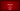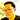# Using Ruby Structs To Subclass

December 10, 2012A struct is Ruby’s convenient way of letting you bundle a bunch of attributes together (complete with accessor methods) without having to define a specific class.

For example I could have:

``````Employee = Struct.new(:name,:id,:company)
puts "Employee is a class?: "+Employee.is_a?(Class).to_s # =>true
puts "Josh is an Employee? "+josh.is_a?(Employee).to_s # =>true``````

With the above, I have defined an Employee class of which josh is an instance.

## Subclassing with Struct

In computer science lingo, a subclass is a class that inherits data and properties from another class. Since a Struct is a class in Ruby, we can subclass on it.

## But why would we want to do this?

One interesting use case I found is to use it to shorten up class definitions. For instance, here is how you would normally define a class:

### Example #1 OriginalCircle Class (normal class definition)

``````class OriginalCircle
def initialize(r)
end

def diameter
end

def circle_area
end
end``````

And here is how you would do it using Ruby’s Struct:

### Example #2 Circle Class (subclassing with struct)

``````class Circle < Struct.new(:radius)
def diameter
end

def circle_area
end
end``````

Notice that by subclassing with a struct (example 2), the “initialize” method is given to you “automagically.”

### You also get the accessor method free of charge

So you can do:

``````c=Circle.new(1)